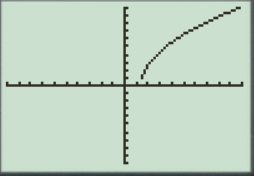# To graph : The quadratic equation y = 12 x − 17 in a graphing window.### Precalculus: Mathematics for Calcu...

6th Edition
Stewart + 5 others
Publisher: Cengage Learning
ISBN: 9780840068071### Precalculus: Mathematics for Calcu...

6th Edition
Stewart + 5 others
Publisher: Cengage Learning
ISBN: 9780840068071

#### Solutions

Chapter 1.9, Problem 16E
To determine

## To graph: The quadratic equation y=12x−17 in a graphing window.

Expert Solution

### Explanation of Solution

Given information:

Graph:

The graph of the quadratic equation y=12x17 can be sketched in the cartesian plane,

Consider the quadratic equation, y=12x17 .

Now put the values of x in the equation to find the values of y or to find the real roots of y with the help of x .

Rewrite the equation:

y=12x17

Take power 2 both sides:

y=12x17y2=12x170=y212x+17

Solve the equation 0=y212x+17 by replace the value of y as zero.

y212x+17=012x+17=012x=17x=1712

Now take y=1 in the equation y2=12x17

y212x+17=01212x+17=0112x=17x=1812

From here the value of x is:

x=1812x=32

Observe that as the value of y increases there is slightly decrement in value of x .

Steps to plot the graph of the equation y2=12x17 with the help of graphing utility are as follows:

Step 1: Press MODE key.

Step 2: Use the down arrow key to reach FUNC option.

Step 3: Press ENTER key.

Step 4: Press Y= key.

Step 5: Enter the function y2=12x17 .

Step 6: Press WINDOW key. Change the settings to

Xmin=20Xmax=10Ymin=10Ymax=20

For better view of graph.

Step 8: Press GRAPH key.

The result obtained on the screen is provided below,Interpretation:

The equation of the function y2=12x17 represents a parabola.

The parabola opens downwards.

The x intercepts are the points on xaxis where the graph of the equation touches xaxis .

At x -axis y is always zero.

Recall that the graphical approach to solve the equation simultaneously.

Therefore, in the equation, y2=12x17 there is no x intercepts.

Therefore, the equation y2=12x17 is symmetric about the y axis Initially the graph of the function y2=12x17 increases when increase the value of x ,the graph is a parabola shaped graph.

### Have a homework question?

Subscribe to bartleby learn! Ask subject matter experts 30 homework questions each month. Plus, you’ll have access to millions of step-by-step textbook answers!